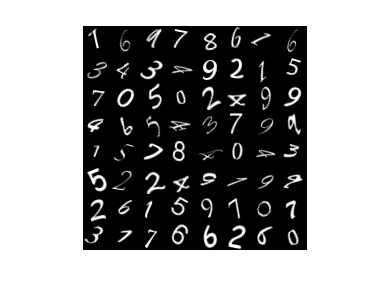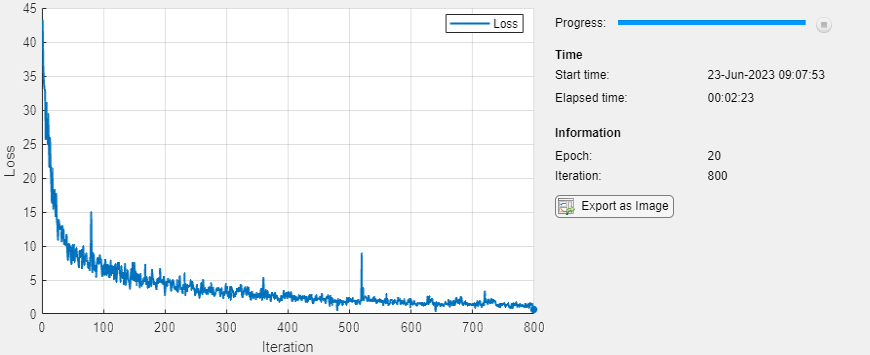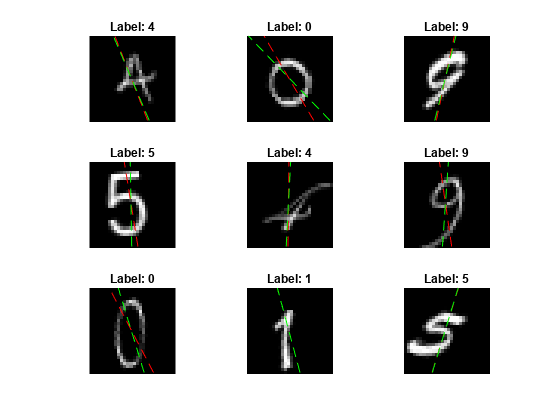# Update Batch Normalization Statistics Using Model Function

This example shows how to update the network state in a network defined as a function.

A batch normalization operation normalizes each input channel across a mini-batch. To speed up training of convolutional neural networks and reduce the sensitivity to network initialization, use batch normalization operations between convolutions and nonlinearities, such as ReLU layers.

During training, batch normalization operations first normalize the activations of each channel by subtracting the mini-batch mean and dividing by the mini-batch standard deviation. Then, the operation shifts the input by a learnable offset β and scales it by a learnable scale factor γ.

When you use a trained network to make predictions on new data, the batch normalization operations use the trained data set mean and variance instead of the mini-batch mean and variance to normalize the activations.

To compute the data set statistics, you must keep track of the mini-batch statistics by using a continually updating state.

If you use batch normalization operations in a model function, then you must define the behavior for both training and prediction. For example, you can specify a Boolean option `doTraining` to control whether the model uses mini-batch statistics for training or data set statistics for prediction.

This example piece of code from a model function shows how to apply a batch normalization operation and update only the data set statistics during training.

```if doTraining [dlY,trainedMean,trainedVariance] = batchnorm(dlY,offset,scale,trainedMean,trainedVariance); % Update state state.batchnorm1.TrainedMean = trainedMean; state.batchnorm1.TrainedVariance = trainedVariance; else dlY = batchnorm(dlY,offset,scale,trainedMean,trainedVariance); end ```

The `digitTrain4DArrayData` function loads the images, their digit labels, and their angles of rotation from the vertical. Create an `arrayDatastore` object for the images, labels, and the angles, and then use the `combine` function to make a single datastore that contains all of the training data. Extract the class names and number of nondiscrete responses.

```[XTrain,YTrain,anglesTrain] = digitTrain4DArrayData; dsXTrain = arrayDatastore(XTrain,'IterationDimension',4); dsYTrain = arrayDatastore(YTrain); dsAnglesTrain = arrayDatastore(anglesTrain); dsTrain = combine(dsXTrain,dsYTrain,dsAnglesTrain); classNames = categories(YTrain); numClasses = numel(classNames); numResponses = size(anglesTrain,2); numObservations = numel(YTrain);```

View some images from the training data.

```idx = randperm(numObservations,64); I = imtile(XTrain(:,:,:,idx)); figure imshow(I)```### Define Deep Learning Model

Define the following network that predicts both labels and angles of rotation.

• A convolution-batchnorm-ReLU block with 16 5-by-5 filters.

• A branch of two convolution-batchnorm blocks each with 32 3-by-3 filters with a ReLU operation between

• A skip connection with a convolution-batchnorm block with 32 1-by-1 convolutions.

• Combine both branches using addition followed by a ReLU operation

• For the regression output, a branch with a fully connected operation of size 1 (the number of responses).

• For classification output, a branch with a fully connected operation of size 10 (the number of classes) and a softmax operation.#### Define and Initialize Model Parameters and State

Define the parameters for each of the operations and include them in a struct. Use the format `parameters.OperationName.ParameterName` where `parameters` is the struct, O`perationName` is the name of the operation (for example "conv1") and `ParameterName` is the name of the parameter (for example, "Weights").

Create a struct `parameters` containing the model parameters. Initialize the learnable layer weights and biases using the `initializeGlorot` and `initializeZeros` example functions, respectively. Initialize the batch normalization offset and scale parameters with the `initializeZeros` and `initializeOnes` example functions, respectively.

To perform training and inference using batch normalization layers, you must also manage the network state. Before prediction, you must specify the dataset mean and variance derived from the training data. Create a struct `state` containing the state parameters. The batch normalization statistics must not be `dlarray` objects. Initialize the batch normalization trained mean and trained variance states using the `zeros` and `ones` functions, respectively.

The initialization example functions are attached to this example as supporting files.

Initialize the parameters for the first convolutional layer.

```filterSize = [5 5]; numChannels = 1; numFilters = 16; sz = [filterSize numChannels numFilters]; numOut = prod(filterSize) * numFilters; numIn = prod(filterSize) * numFilters; parameters.conv1.Weights = initializeGlorot(sz,numOut,numIn); parameters.conv1.Bias = initializeZeros([numFilters 1]);```

Initialize the parameters and state for the first batch normalization layer.

```parameters.batchnorm1.Offset = initializeZeros([numFilters 1]); parameters.batchnorm1.Scale = initializeOnes([numFilters 1]); state.batchnorm1.TrainedMean = zeros(numFilters,1,'single'); state.batchnorm1.TrainedVariance = ones(numFilters,1,'single');```

Initialize the parameters for the second convolutional layer.

```filterSize = [3 3]; numChannels = 16; numFilters = 32; sz = [filterSize numChannels numFilters]; numOut = prod(filterSize) * numFilters; numIn = prod(filterSize) * numFilters; parameters.conv2.Weights = initializeGlorot(sz,numOut,numIn); parameters.conv2.Bias = initializeZeros([numFilters 1]);```

Initialize the parameters and state for the second batch normalization layer.

```parameters.batchnorm2.Offset = initializeZeros([numFilters 1]); parameters.batchnorm2.Scale = initializeOnes([numFilters 1]); state.batchnorm2.TrainedMean = zeros(numFilters,1,'single'); state.batchnorm2.TrainedVariance = ones(numFilters,1,'single');```

Initialize the parameters for the third convolutional layer.

```filterSize = [3 3]; numChannels = 32; numFilters = 32; sz = [filterSize numChannels numFilters]; numOut = prod(filterSize) * numFilters; numIn = prod(filterSize) * numFilters; parameters.conv3.Weights = initializeGlorot(sz,numOut,numIn); parameters.conv3.Bias = initializeZeros([numFilters 1]);```

Initialize the parameters and state for the third batch normalization layer.

```parameters.batchnorm3.Offset = initializeZeros([numFilters 1]); parameters.batchnorm3.Scale = initializeOnes([numFilters 1]); state.batchnorm3.TrainedMean = zeros(numFilters,1,'single'); state.batchnorm3.TrainedVariance = ones(numFilters,1,'single');```

Initialize the parameters for the convolutional layer in the skip connection.

```filterSize = [1 1]; numChannels = 16; numFilters = 32; sz = [filterSize numChannels numFilters]; numOut = prod(filterSize) * numFilters; numIn = prod(filterSize) * numFilters; parameters.convSkip.Weights = initializeGlorot(sz,numOut,numIn); parameters.convSkip.Bias = initializeZeros([numFilters 1]);```

Initialize the parameters and state for the batch normalization layer in the skip connection.

```parameters.batchnormSkip.Offset = initializeZeros([numFilters 1]); parameters.batchnormSkip.Scale = initializeOnes([numFilters 1]); state.batchnormSkip.TrainedMean = zeros([numFilters 1],'single'); state.batchnormSkip.TrainedVariance = ones([numFilters 1],'single');```

Initialize the parameters for the fully connected layer corresponding to the classification output.

```sz = [numClasses 6272]; numOut = numClasses; numIn = 6272; parameters.fc1.Weights = initializeGlorot(sz,numOut,numIn); parameters.fc1.Bias = initializeZeros([numClasses 1]);```

Initialize the parameters for the fully connected layer corresponding to the regression output.

```sz = [numResponses 6272]; numOut = numResponses; numIn = 6272; parameters.fc2.Weights = initializeGlorot(sz,numOut,numIn); parameters.fc2.Bias = initializeZeros([numResponses 1]);```

View the struct of the state.

`state`
```state = struct with fields: batchnorm1: [1×1 struct] batchnorm2: [1×1 struct] batchnorm3: [1×1 struct] batchnormSkip: [1×1 struct] ```

View the state parameters for the `batchnorm1` operation.

`state.batchnorm1`
```ans = struct with fields: TrainedMean: [16×1 single] TrainedVariance: [16×1 single] ```

#### Define Model Function

Create the function `model`, listed at the end of the example, which computes the outputs of the deep learning model described earlier.

The function `model` takes as input the model parameters `parameters`, input data `dlX`, the flag `doTraining`, which specifies whether the model returns outputs for training or prediction, and the network state `state`. The network outputs the predictions for the labels, the predictions for the angles, and the updated network state.

Create the function `modelGradients`, listed at the end of the example, which takes as input a mini-batch of input data `dlX` with corresponding targets `T1` and `T2` containing the labels and angles, respectively, and returns the gradients of the loss with respect to the learnable parameters, the updated network state, and the corresponding loss.

### Specify Training Options

Specify the training options.

```numEpochs = 20; miniBatchSize = 128; plots = "training-progress";```

### Train Model

Train the model using a custom training loop. Use `minibatchqueue` to process and manage the mini-batches of images. For each mini-batch:

• Use the custom mini-batch preprocessing function `preprocessMiniBatch` (defined at the end of this example) to one-hot encode the class labels.

• Format the image data with the dimension labels `'SSCB'` (spatial, spatial, channel, batch). By default, the `minibatchqueue` object converts the data to `dlarray` objects with underlying type `single`. Do not add a format to the class labels or the angles.

• Train on a GPU if one is available. By default, the `minibatchqueue` object converts each output to a `gpuArray` if a GPU is available. Using a GPU requires Parallel Computing Toolbox™ and a supported GPU device. For information on supported devices, see GPU Support by Release (Parallel Computing Toolbox).

```mbq = minibatchqueue(dsTrain,... 'MiniBatchSize',miniBatchSize,... 'MiniBatchFcn', @preprocessMiniBatch,... 'MiniBatchFormat',{'SSCB','',''});```

For each epoch, shuffle the data and loop over mini-batches of data. At the end of each epoch, display the training progress. For each mini-batch:

• Evaluate the model gradients and loss using `dlfeval` and the `modelGradients` function.

• Update the network parameters using the `adamupdate` function.

Initialize the parameters for the Adam solver.

```trailingAvg = []; trailingAvgSq = [];```

Initialize the training progress plot.

```if plots == "training-progress" figure lineLossTrain = animatedline('Color',[0.85 0.325 0.098]); ylim([0 inf]) xlabel("Iteration") ylabel("Loss") grid on end```

Train the model.

```iteration = 0; start = tic; % Loop over epochs. for epoch = 1:numEpochs % Shuffle data. shuffle(mbq) % Loop over mini-batches while hasdata(mbq) iteration = iteration + 1; [dlX,dlY1,dlY2] = next(mbq); % Evaluate the model gradients, state, and loss using dlfeval and the % modelGradients function. [gradients,state,loss] = dlfeval(@modelGradients, parameters, dlX, dlY1, dlY2, state); % Update the network parameters using the Adam optimizer. [parameters,trailingAvg,trailingAvgSq] = adamupdate(parameters,gradients, ... trailingAvg,trailingAvgSq,iteration); % Display the training progress. if plots == "training-progress" D = duration(0,0,toc(start),'Format','hh:mm:ss'); addpoints(lineLossTrain,iteration,double(gather(extractdata(loss)))) title("Epoch: " + epoch + ", Elapsed: " + string(D)) drawnow end end end```### Test Model

Test the classification accuracy of the model by comparing the predictions on a test set with the true labels and angles. Manage the test data set using a `minibatchqueue` object with the same setting as the training data.

```[XTest,Y1Test,anglesTest] = digitTest4DArrayData; dsXTest = arrayDatastore(XTest,'IterationDimension',4); dsYTest = arrayDatastore(Y1Test); dsAnglesTest = arrayDatastore(anglesTest); dsTest = combine(dsXTest,dsYTest,dsAnglesTest); mbqTest = minibatchqueue(dsTest,... 'MiniBatchSize',miniBatchSize,... 'MiniBatchFcn', @preprocessMiniBatch,... 'MiniBatchFormat',{'SSCB','',''});```

To predict the labels and angles of the validation data, use the `modelPredictions` function, listed at the end of the example. The function returns the predicted classes and angles, as well as comparison with the true values.

`[classesPredictions,anglesPredictions,classCorr,angleDiff] = modelPredictions(parameters,state,mbqTest,classNames);`

Evaluate the classification accuracy.

`accuracy = mean(classCorr)`
```accuracy = 0.9840 ```

Evaluate the regression accuracy.

`angleRMSE = sqrt(mean(angleDiff.^2))`
```angleRMSE = single 6.3669 ```

View some of the images with their predictions. Display the predicted angles in red and the correct angles in green.

```idx = randperm(size(XTest,4),9); figure for i = 1:9 subplot(3,3,i) I = XTest(:,:,:,idx(i)); imshow(I) hold on sz = size(I,1); offset = sz/2; thetaPred = anglesPredictions(idx(i)); plot(offset*[1-tand(thetaPred) 1+tand(thetaPred)],[sz 0],'r--') thetaValidation = anglesTest(idx(i)); plot(offset*[1-tand(thetaValidation) 1+tand(thetaValidation)],[sz 0],'g--') hold off label = string(classesPredictions(idx(i))); title("Label: " + label) end```### Model Function

The function `model` takes as input the model parameters `parameters`, the input data `dlX`, the flag `doTraining`, which specifies whether the model returns the outputs for training or prediction, and the network state `state`. The function returns the predictions for the labels, the predictions for the angles, and the updated network state.

```function [dlY1,dlY2,state] = model(parameters,dlX,doTraining,state) % Convolution weights = parameters.conv1.Weights; bias = parameters.conv1.Bias; dlY = dlconv(dlX,weights,bias,'Padding',2); % Batch normalization, ReLU offset = parameters.batchnorm1.Offset; scale = parameters.batchnorm1.Scale; trainedMean = state.batchnorm1.TrainedMean; trainedVariance = state.batchnorm1.TrainedVariance; if doTraining [dlY,trainedMean,trainedVariance] = batchnorm(dlY,offset,scale,trainedMean,trainedVariance); % Update state state.batchnorm1.TrainedMean = trainedMean; state.batchnorm1.TrainedVariance = trainedVariance; else dlY = batchnorm(dlY,offset,scale,trainedMean,trainedVariance); end dlY = relu(dlY); % Convolution, batch normalization (skip connection) weights = parameters.convSkip.Weights; bias = parameters.convSkip.Bias; dlYSkip = dlconv(dlY,weights,bias,'Stride',2); offset = parameters.batchnormSkip.Offset; scale = parameters.batchnormSkip.Scale; trainedMean = state.batchnormSkip.TrainedMean; trainedVariance = state.batchnormSkip.TrainedVariance; if doTraining [dlYSkip,trainedMean,trainedVariance] = batchnorm(dlYSkip,offset,scale,trainedMean,trainedVariance); % Update state state.batchnormSkip.TrainedMean = trainedMean; state.batchnormSkip.TrainedVariance = trainedVariance; else dlYSkip = batchnorm(dlYSkip,offset,scale,trainedMean,trainedVariance); end % Convolution weights = parameters.conv2.Weights; bias = parameters.conv2.Bias; dlY = dlconv(dlY,weights,bias,'Padding',1,'Stride',2); % Batch normalization, ReLU offset = parameters.batchnorm2.Offset; scale = parameters.batchnorm2.Scale; trainedMean = state.batchnorm2.TrainedMean; trainedVariance = state.batchnorm2.TrainedVariance; if doTraining [dlY,trainedMean,trainedVariance] = batchnorm(dlY,offset,scale,trainedMean,trainedVariance); % Update state state.batchnorm2.TrainedMean = trainedMean; state.batchnorm2.TrainedVariance = trainedVariance; else dlY = batchnorm(dlY,offset,scale,trainedMean,trainedVariance); end dlY = relu(dlY); % Convolution weights = parameters.conv3.Weights; bias = parameters.conv3.Bias; dlY = dlconv(dlY,weights,bias,'Padding',1); % Batch normalization offset = parameters.batchnorm3.Offset; scale = parameters.batchnorm3.Scale; trainedMean = state.batchnorm3.TrainedMean; trainedVariance = state.batchnorm3.TrainedVariance; if doTraining [dlY,trainedMean,trainedVariance] = batchnorm(dlY,offset,scale,trainedMean,trainedVariance); % Update state state.batchnorm3.TrainedMean = trainedMean; state.batchnorm3.TrainedVariance = trainedVariance; else dlY = batchnorm(dlY,offset,scale,trainedMean,trainedVariance); end % Addition, ReLU dlY = dlYSkip + dlY; dlY = relu(dlY); % Fully connect, softmax (labels) weights = parameters.fc1.Weights; bias = parameters.fc1.Bias; dlY1 = fullyconnect(dlY,weights,bias); dlY1 = softmax(dlY1); % Fully connect (angles) weights = parameters.fc2.Weights; bias = parameters.fc2.Bias; dlY2 = fullyconnect(dlY,weights,bias); end```

The `modelGradients` function takes as input the model parameters, a mini-batch of the input data `dlX` with corresponding targets `T1` and `T2` containing the labels and angles, respectively, and returns the gradients of the loss with respect to the learnable parameters, the updated network state, and the corresponding loss.

```function [gradients,state,loss] = modelGradients(parameters,dlX,T1,T2,state) doTraining = true; [dlY1,dlY2,state] = model(parameters,dlX,doTraining,state); lossLabels = crossentropy(dlY1,T1); lossAngles = mse(dlY2,T2); loss = lossLabels + 0.1*lossAngles; gradients = dlgradient(loss,parameters); end```

### Model Predictions Function

The `modelPredictions` function takes the model parameters, the network state, a `minibatchqueue` of input data `mbq`, and the network classes, and returns the model predictions by iterating over all data in the `minibatchqueue` using the `model` function with the `doTraining` option set to `false`. The function returns the predicted classes and angles, as well as comparison with the true values. For the classes, the comparison is a vector of ones and zeros that represents correct and incorrect predictions. For the angles, the comparison is the difference between the predicted angle and the true value.

```function [classesPredictions,anglesPredictions,classCorr,angleDiff] = modelPredictions(parameters,state,mbq,classes) doTraining = false; classesPredictions = []; anglesPredictions = []; classCorr = []; angleDiff = []; while hasdata(mbq) [dlX,dlY1,dlY2] = next(mbq); % Make predictions using the model function. [dlY1Pred,dlY2Pred] = model(parameters,dlX,doTraining,state); % Determine predicted classes. Y1PredBatch = onehotdecode(dlY1Pred,classes,1); classesPredictions = [classesPredictions Y1PredBatch]; % Dermine predicted angles Y2PredBatch = extractdata(dlY2Pred); anglesPredictions = [anglesPredictions Y2PredBatch]; % Compare predicted and true classes Y1 = onehotdecode(dlY1,classes,1); classCorr = [classCorr Y1PredBatch == Y1]; % Compare predicted and true angles angleDiffBatch = Y2PredBatch - dlY2; angleDiff = [angleDiff extractdata(gather(angleDiffBatch))]; end end```

### Mini-Batch Preprocessing Function

The `preprocessMiniBatch` function preprocesses the data using the following steps:

1. Extract the image data from the incoming cell array and concatenate into a numeric array. Concatenating the image data over the fourth dimension adds a third dimension to each image, to be used as a singleton channel dimension.

2. Extract the label and angle data from the incoming cell arrays and concatenate into a categorical array and a numeric array, respectively.

3. One-hot encode the categorical labels into numeric arrays. Encoding into the first dimension produces an encoded array that matches the shape of the network output.

```function [X,Y,angle] = preprocessMiniBatch(XCell,YCell,angleCell) % Extract image data from cell and concatenate X = cat(4,XCell{:}); % Extract label data from cell and concatenate Y = cat(2,YCell{:}); % Extract angle data from cell and concatenate angle = cat(2,angleCell{:}); % One-hot encode labels Y = onehotencode(Y,1); end```